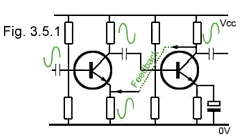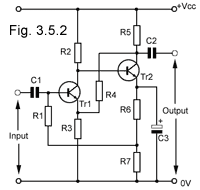# Negative Feedback Quiz

Try our quiz, based on the information you can find in Amplifiers Module 3. Submit your answers and see how many you get right. If you get any answers wrong. Just follow the hints to find the right answer and learn about Amplifiers as you go.

### 1.

Which of the following correctly lists the benefits of using NFB in an amplifier system?

### 2.

If a voltage amplifier has an open loop gain (AO) of 150 and a feedback factor (β) of 1/20, what will be the approximate gain with negative feedback applied?

### 3.

What is the NFB method being used in Fig. 3.5.1?### 4.

What is the main advantage of using direct coupling in multi stage NFB amplifiers?

### 5.

Refer to Fig. 3.5.2: Which of the following statements most accurately describes the type of inter stage coupling and method of feedback used?### 6.

Refer to Fig. 3.5.2: Assuming that the input signal is normal, what would be the probable effect on the circuit if R4 became open circuit?

### 7.

To increase the input impedance, and reduce the output impedance of a multi stage amplifier, which of the following NFB methods should be used?

### 8.

In an audio amplifier using NFB which of the following methods would be most effective in reducing electro-magnetically induced noise from the power supply?

### 9.

Negative feedback loops avoid the use of reactive components where possible to minimise which of the following effects?

### 10.

The audio amplifier response curve illustrated in Fig. 3.5.3 shows which of the following problems?Top of Page